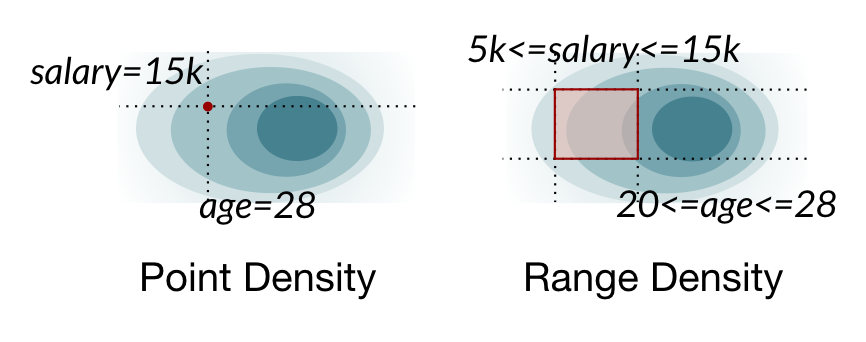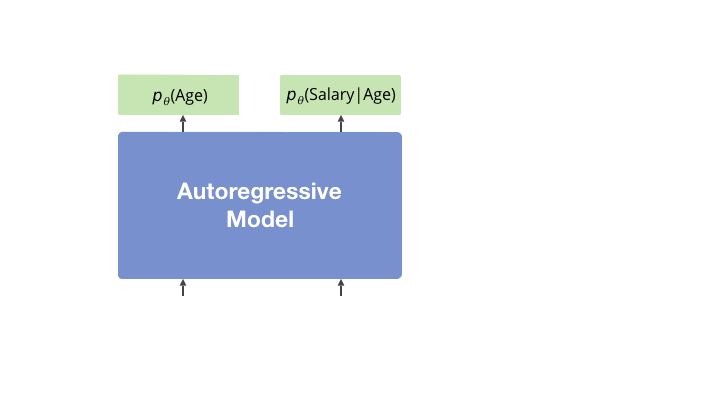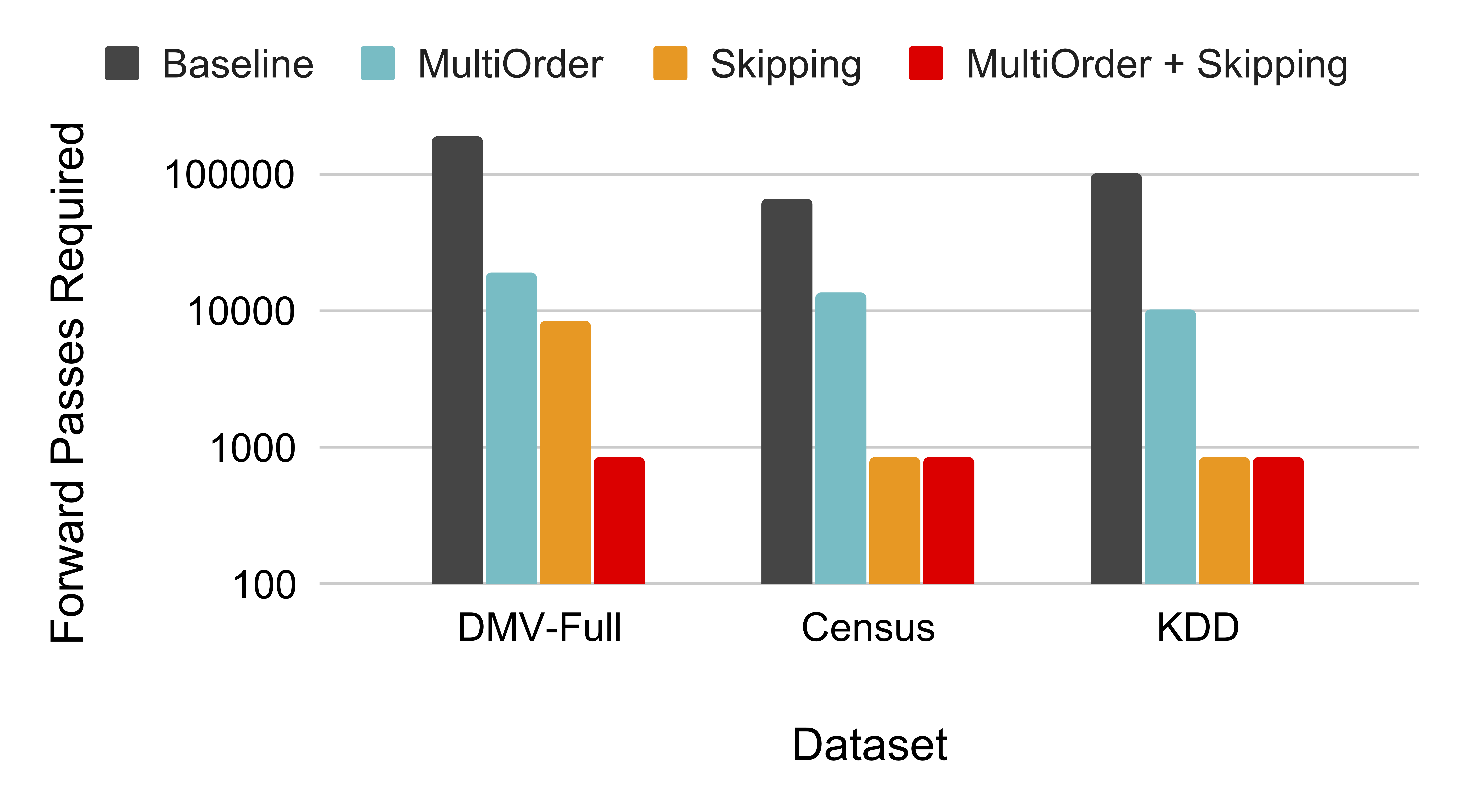# Variable Skipping for Autoregressive Range Density Estimation

##### ICML 2020Point vs. range density estimation. Naive marginalization to estimate range densities takes time exponential in the number of dimensions of the joint distribution.

## Summary

• We propose range density estimation, a new downstream task for autoregressive models.
• Inspired by ML-for-systems application: database query optimization.
• Prior work uses a forward sampling-style algorithm for approximate inference.
• We speed up inference by simple data augmentation: randomly mask inputs at training; invoke learned masks to skip inferring unconstrained variables at inference.
• Variable skipping outperforms multi-order training alone, and can provide additional gains when used in combination.
• We invite future research on this relatively under-explored task, where better performance translates to real-world impact (faster query engines).

## Abstract

Deep autoregressive models compute point likelihood estimates of individual data points. However, many applications (i.e., database cardinality estimation) require estimating range densities, a capability that is under-explored by current neural density estimation literature. In these applications, fast and accurate range density estimates over high-dimensional data directly impact user-perceived performance. In this paper, we explore a technique, variable skipping, for accelerating range density estimation over deep autoregressive models. This technique exploits the sparse structure of range density queries to avoid sampling unnecessary variables during approximate inference. We show that variable skipping provides 10-100x efficiency improvements when targeting challenging high-quantile error metrics, enables complex applications such as text pattern matching, and can be realized via a simple data augmentation procedure without changing the usual maximum likelihood objective.

## The Range Density Estimation Task

We consider an autoregressive model trained on a high-dimensional discrete dataset. Range density queries require the following probability: $$p_\theta(X_1 \in R_1, \dots, X_n \in R_n)$$where each region $R_i$ is a subset of variable $i$'s domain.

Example: database queries. SQL queries often contain such range/region constraints on columns. Here's an example query on a personnel database:

SELECT * FROM personnel WHERE salary > 5000 AND age = 28

This corresponds to $p_\theta(\text{salary} > 5000, \text{age} = 28)$, possibly leaving out unconstrained variables. Database optimizers require these estimates to make queries go fast.

Other applications. In the paper, we show this task is important for another application, text pattern matching: e.g., what's the match probability of .*icml.* in a corpus?

Approximate inference and unconstrained variables. Exact inference of the range query incurs an exponential cost. Naru (VLDB 2020) instead adapts forward sampling to perform approximate inference (Algorithm 1).

Our insight is to exploit the inherent sparsity in queries: they often access just a few columns out of many in the dataset (e.g., imagine education, city, zip in the above database, which are unconstrained). Such variables should not unnecessarily contribute to the cost of the sampling-based approximate inference.

## Variable Skipping

To skip sampling unconstrained/absent variables during inference, we propose training special MASK tokens that signify a variable's absence:MASK positions are sampled uniformly (see paper for ablation details).

When inferring a range query, we directly marginalize all unconstrained variables by invoking these tokens: i.e., each unconstrained variable $i$ is set to its marginalization token, $X_i = \textsf{MASK}_i$.

## Comparison with Masked Language Modeling (MLM)

Masking has been used in related contexts.

BERT's masked language model (MLM) objective optimizes for $\prod_{i \in \text{masked}} p_\theta(X_{_i} | X_\text{unmasked})$, which (i) only predicts the masked tokens and (ii) assumes the masked tokens are independent. Typically, the learned masks are not used downstream.

In contrast, variable skipping's training objective is still autoregressive: $$\prod_{\forall i} p_\theta(X_{i}\, |\, \text{RandomMask}(X_{\\< i}))$$ i.e., the network is asked to predict all tokens, conditioning on a mix of present and absent (masked) prior information. This can be viewed as input dropout or a form of data augmentation.

Lastly, variable skipping uses the learned masked tokens in the downstream task to signify the marginalization of variables.

## Results

We evaluate on discrete tabular datasets, using 1,000 randomly generated range queries. Variable skipping provides 10-100x compute savings for challenging high-quantile error targets compared to baseline approximate inference.Approximate number of model forward passes required to achieve single-digit inference error at the 99th quantile.

Compared with multi-order training, where each model performs inference using an ensemble of orders, variable skipping outperforms it by up to 10x. It can provide additional gains when used in combination on the more challenging dataset (DMV-Full).

Refer to the paper for more results (variable skipping + Transformer for pattern matching; ablation studies).

## Citation

@inproceedings{varskip,  title={Variable Skipping for Autoregressive Range Density Estimation},  author={Eric Liang and Zongheng Yang and Ion Stoica and Pieter Abbeel and Yan Duan and Xi Chen},  year={2020},  booktitle={Proceedings of the 37th International Conference on Machine Learning}}

Page style file credited to ContraCode.